Next: Multi-Dimensional Search (No Up: Theory: Optimization Methods Previous: Golden Section Search

## Multi-Dimensional Search

Finding the n parameters a which minimize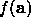when n>1 is a much harder problem. The n=2 case is like trying to find the point at the bottom of the lowest valley in a range of mountains. Higher dimensional cases are hard to visualize at all. This author usually just pretends they are 2-D problems and tries not to worry too much.

When presented with a new problem it is usually fruitful to get a feel for the shape of the function surface by plottingagainst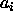for each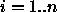, in each case keeping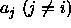fixed.

As mentioned above the choice of local minimizer will depend upon whether it is possible to generate first or second derivatives efficiently, and how noisy the function is. All the local minimizers below assume that the function is locally relatively smooth - any noise will confuse the derivative estimates and may confound the algorithm. For such functions the Simplex Method is recommended (Section 2.7.1).

Most of the local minimizers below rely on approximating the function about the current point, a, using a Taylor series expansion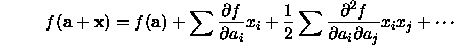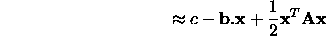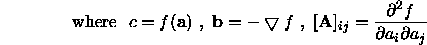The matrix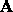is called the Hessian matrix of the function at a.

At the minimum the gradient is zero (if it wasn't we could move further downhill), so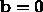and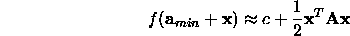(where A is the Hessian evaluated at the minimum point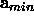).